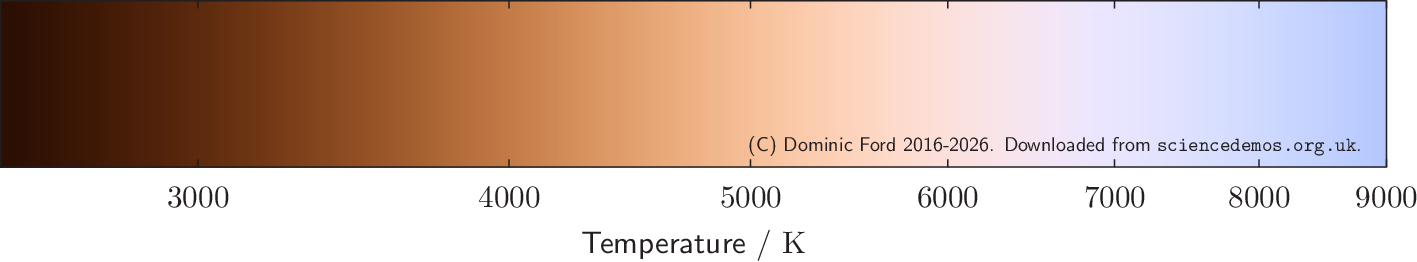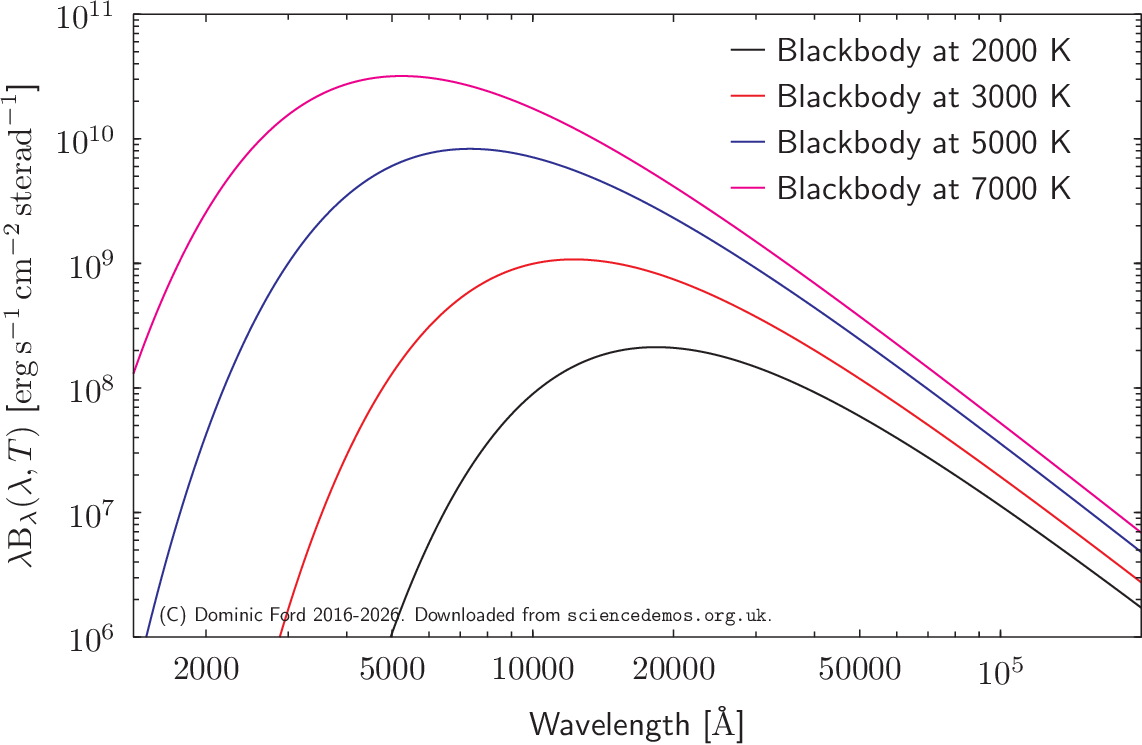The color of blackbodies

by Dominic FordThe colors of blackbodies as a function of temperature. Download image as pdf | png.

The tool above converts any specified temperature of blackbody into the RGB color which most closely approximates the color of the light emitted by such a blackbody.

Blackbody emission is the "thermal" emission that objects emit when they become "red hot". Objects that are cooler than around 2000°C emit exclusively in the infrared, at wavelengths longer than the human eye can perceive, but when an object becomes red hot, its emission begins to reach into the red end of the visible spectrum of light.

The term "blackbody" simply refers to an object with no intrinsic coloration of its surface. In practice, objects often have colored surfaces which make them absorb and emit light at some wavelengths more preferentially to other wavelengths. This modifies the precise spectrum of light they reflect and emit.The spectral energy distributions (SEDs) of blackbodies as a function of temperature. Download image as pdf | png.

According to Planck's law, the light emitted by a blackbody has the spectral energy distribution (SED) – i.e. intensity as a function of wavelength – shown to the right, given by the formula:

$\displaystyle B_{\lambda }(\lambda ,T)={\frac {2hc^{2}}{\lambda ^{5}}}{\frac {1}{e^{ hc / (\lambda k_{\mathrm {B} }T)}-1}}$

To convert this into a RGB color which can be represented on a computer display, we use the method described in the Drawing the electromagnetic spectrum demo. However, instead of simply working out the RGB color which represents a single wavelength of light, we add together the RGB colors of all the wavelengths of light within the blackbody spectrum.